# Principles of Marketing - Philip Kotler

## Calculation of key ratios

Report on the results of operations contains the data required to derive a number of key factors. Typically, this so-called operating factors, i.e. percentages of individual indicators to report the amount of net sales, which give entrepreneurs the opportunity to compare the achievements of this year, with the results of the previous (or competitors indicators with the sector in the same year) to determine the degree of success of the company. Most often expect these operational factors, as a share of gross profit margin, the share of net income, the share of operating costs, as well as the share of refunds and rebates.

Another useful from the point of view of analytical work coefficient is an indicator of the intensity of inventory turnover. Intensity inventory turnover - is the number of times a complete renewal or complete sale of goods for a certain length of time (usually a year). This rate can be calculated from the sum of the cost of the goods, the amount of the selling price of the goods sold or the number of trade items.

#### RATIO CALCULATION FORMULA

Calculation according to the report

####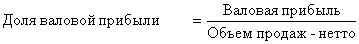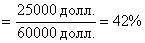####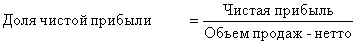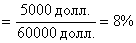####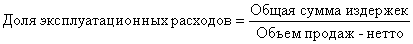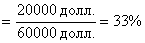####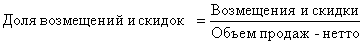The calculation formula may be as follows: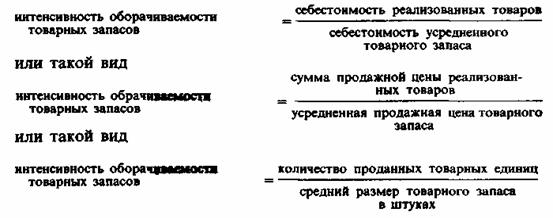The calculation of the first formula: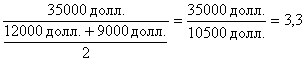This means that the supply of goods in the store Parsons during 1983 completely renewed 3.3 times. Typically, the higher the intensity of inventory turnover, the higher the efficiency of management of the company and its profitability.

As a criterion for evaluating the effectiveness of management are often an indicator of the rate of return on invested capital, which is calculated on the basis of the data contained in the report on the results of operations and balance sheet of the company report. Most often, for the calculation of this index use the following formula:In considering this formula, two questions arise. First, why use a two-stage procedure, if the rate of return on capital can be derived as a simple ratio of net profit to invested capital? And secondly, what exactly is meant by "invested capital"?

The rationale for the answer to the first question can be obtained by finding out how the impact each of the constituent elements of the formula in the rates of return. Suppose Parsons expected rate of return on invested capital as follows:If Parsons believed that the increase in owned his share of the clothing market will give him some marketing advantages he might have achieved the same rate of return on invested capital, doubling the volume of sales at constant levels of income and capital (by reducing the rate of profit at the same time growth of turnover and market share):Increases in the rate of return on invested capital Parsons could be achieved through the growth of net profit by implementing a more perfect marketing plan, its implementation and more effective control:Another way to increase the rate of return on invested capital is to find ways to reduce capital investment (possibly by reducing the average amount of its inventory Parsons), while maintaining previous levels of sales and earnings:And what is meant by "invested capital" in the formula for calculating the rate of return on invested capital? Often people think that the "cost of capital" - is the sum of all the firm's assets. Today, however, many researchers in the evaluation of the effectiveness of the company management take to calculate the rate of return the other original data. Some estimates are rates of return on invested capital in the form of company net assets, the other - in the form of equity, the third - in the form of working capital. Since the volume of investment is measured at a certain time, the rate of return on invested capital is calculated based on the average amount of investment between the two points in time (eg, between 1 January and 31 December of the same year). The rate of return on the invested capital can also count as "internal rules" based on an analysis of future cash receipts given in the assessment of the present time (for details on this method, see. In any textbook on financial problems). The purpose of these measurements is to determine how effectively the firm uses its resources. As inflation, competitive pressures and the cost of capital of such measurements are an important barometer of the effectiveness of marketing and company management.